# What is the Maximum Likelihood Estimation

2018, Aug 26
• likelihood : A probability of happening possibility of an event.

• Bernoulli Distribution :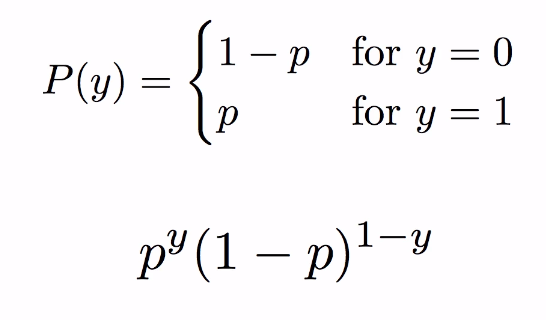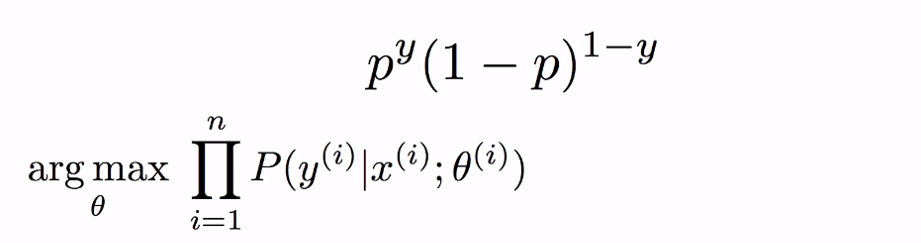In this notation, We calculate the probability given x and theta.
Object is finding θ which maximize the probability.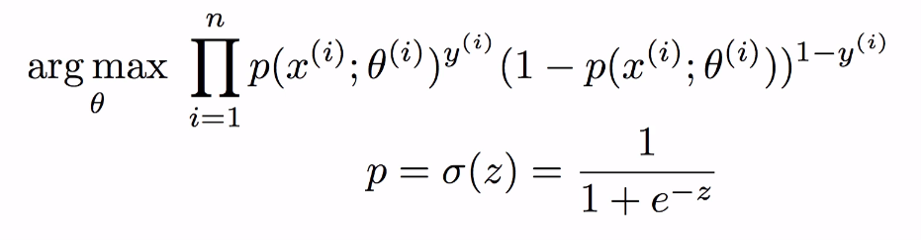In the bernoulli distribution, let me suppose that p is sigmoid.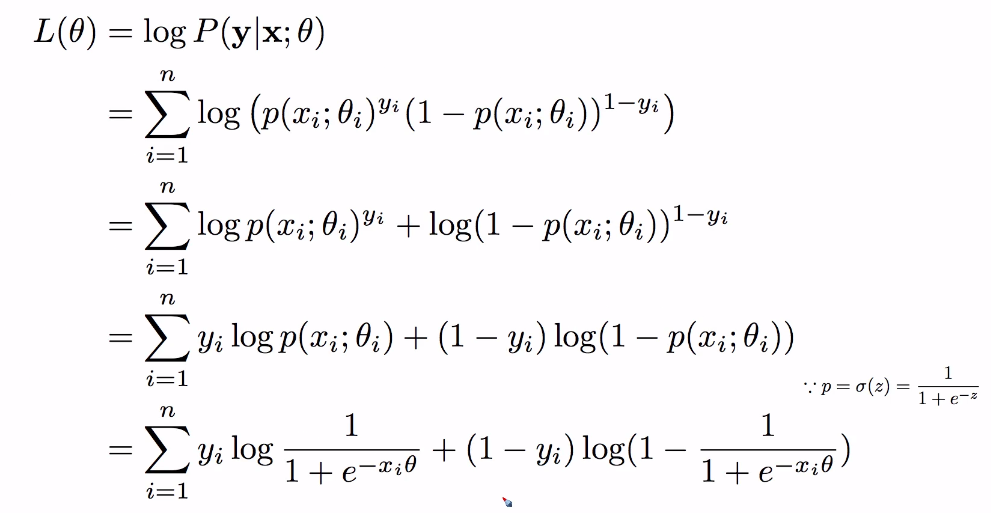And then, we can get likelihood with taking the log function.

Why do we take a log function? Because earlier notation, To get the probability, we multiplied all probabilities.
Multiplying too many probabilities makes the result too much small. Therefore, we transform the multiply to add by log function.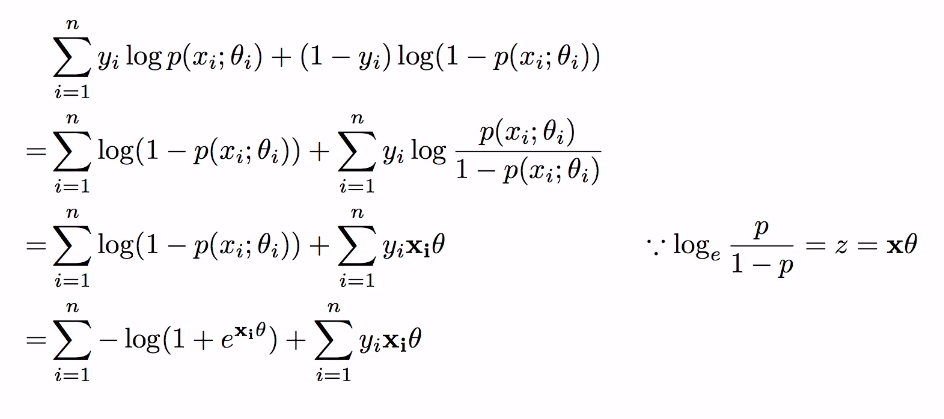,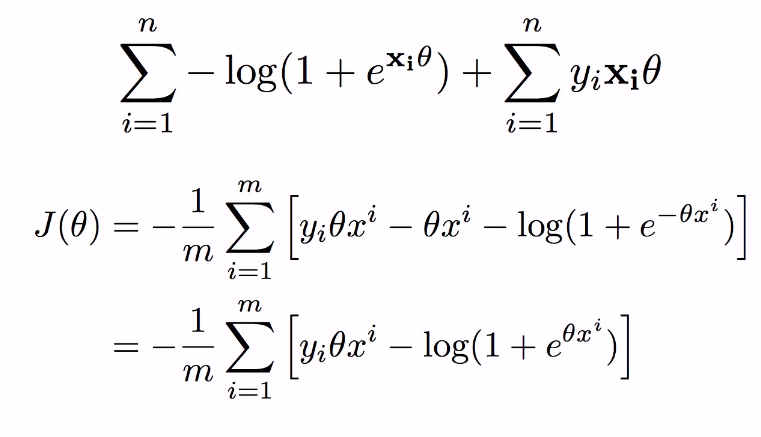Wow, It’s same with cost function of logistic regression.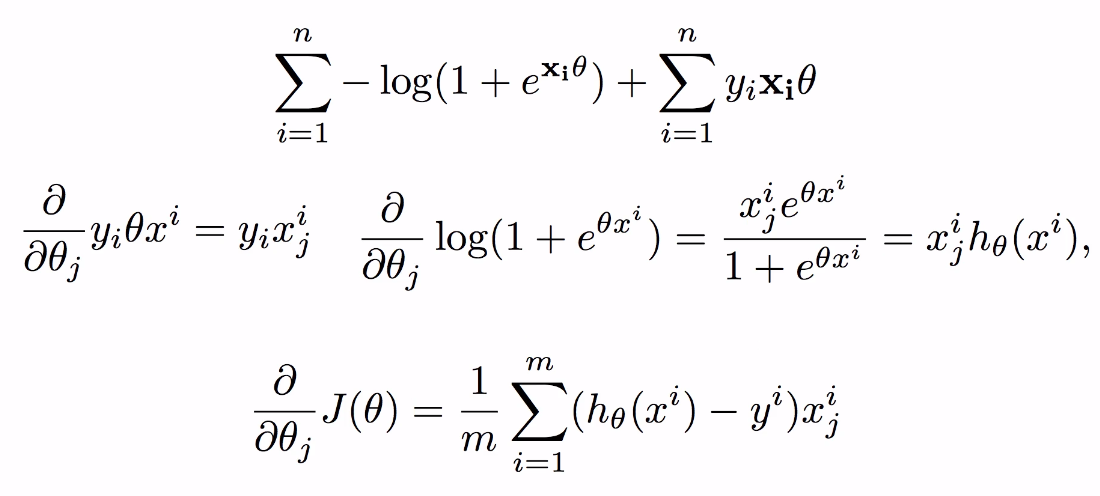Surely, partial derivation of MLE is same with partial derivation of cost function.

### Remember!

• MLE and Cost function have same result in logistic regression !!
• Therefore, we can use cost function as maximize the parameter θ.